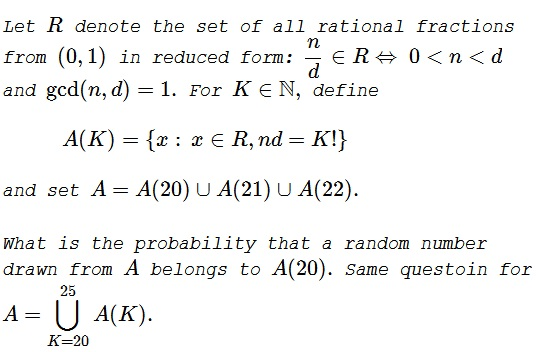# Probability with Factorials

### Problem### Solution

Let's list the prime factors of, say, $20!$ All are less than $20;$ there are eight of them: $2,$ $3,$ $5,$ $7,$ $11,$ $13,$ $17,$ $19.$ Since we are only concerned with the fractions in reduced form, these factors are somehow distributed between their numerators $n$ and denominators $d,$ (in whatever power it takes) but no factor appears in both $n$ and $d.$ Therefore, there are $2^8=256$ possible values for pairs $n,d.$ For only half of them $n\lt d,$ making the number of eligible fractions in $A(20!)$ equal $128.$

Since there are no primes between $19$ and $23,$ $A(21!)$ and $A(22!)$ also have $128$ elements each. Since the pairwise intersections of the three sets are empty, a number drawn from their union has equal probability to be drawn from either of them, i.e., $\displaystyle \frac{1}{3}.$

$23!,$ $24!$ and $25!$ include an additional prime - $23,$ implying that each contains $\displaystyle \frac{1}{2}2^9=256$ elements. Thus, in this case, $|A|=3*128+3*256=9*128.$ Thus the probability of the drawn number to be from $A(20)$ is $\displaystyle \frac{1}{9}.$

### Acknowledgment

This problem was inspired by problem 10 from T. Andreescu and Z. Feng's 102 Combinatorial Problems (Birkhäuser, 2003). The latter was offered at the AIME 1991.